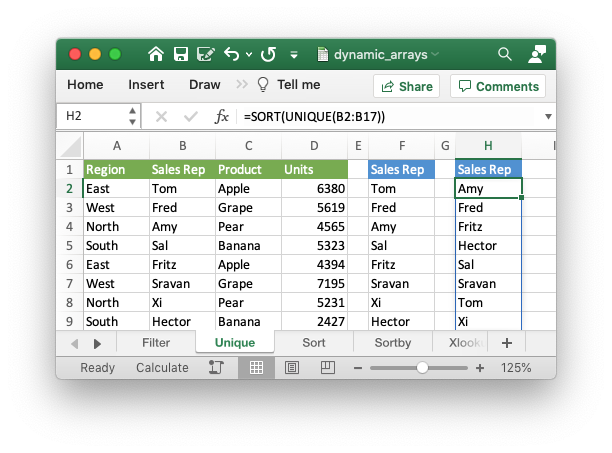# Example: Dynamic array formulas

This program is an example of writing formulas that work with dynamic arrays using some of the new functions and functionality introduced in Excel 365. See the `write_dynamic_array_formula()` method and Dynamic Array support for more details.```#######################################################################
#
# An example of how to use the XlsxWriter module to write formulas and
# functions that create dynamic arrays. These functions are new to Excel
# 365. The examples mirror the examples in the Excel documentation on these
# functions.
#
# Copyright 2013-2023, John McNamara, jmcnamara@cpan.org
#
import xlsxwriter

def main():
# Create a new workbook called simple.xls and add some worksheets.
workbook = xlsxwriter.Workbook('dynamic_arrays.xlsx')

#
# Example of using the FILTER() function.
#
worksheet1.write('F2', '=FILTER(A1:D17,C1:C17=K2)')

# Write the data the function will work on.
worksheet1.write('K2', 'Apple')

worksheet1.set_column_pixels('E:E', 20)
worksheet1.set_column_pixels('J:J', 20)

#
# Example of using the UNIQUE() function.
#
worksheet2.write('F2', '=UNIQUE(B2:B17)')

# A more complex example combining SORT and UNIQUE.
worksheet2.write('H2', '=SORT(UNIQUE(B2:B17))')

# Write the data the function will work on.

worksheet2.set_column_pixels('E:E', 20)
worksheet2.set_column_pixels('G:G', 20)

#
# Example of using the SORT() function.
#
worksheet3.write('F2', '=SORT(B2:B17)')

# A more complex example combining SORT and FILTER.
worksheet3.write('H2', '=SORT(FILTER(C2:D17,D2:D17>5000,""),2,1)')

# Write the data the function will work on.

worksheet3.set_column_pixels('E:E', 20)
worksheet3.set_column_pixels('G:G', 20)

#
# Example of using the SORTBY() function.
#
worksheet4.write('D2', '=SORTBY(A2:B9,B2:B9)')

# Write the data the function will work on.

worksheet4.write('A2', 'Tom')
worksheet4.write('A3', 'Fred')
worksheet4.write('A4', 'Amy')
worksheet4.write('A5', 'Sal')
worksheet4.write('A6', 'Fritz')
worksheet4.write('A7', 'Srivan')
worksheet4.write('A8', 'Xi')
worksheet4.write('A9', 'Hector')

worksheet4.write('B2', 52)
worksheet4.write('B3', 65)
worksheet4.write('B4', 22)
worksheet4.write('B5', 73)
worksheet4.write('B6', 19)
worksheet4.write('B7', 39)
worksheet4.write('B8', 19)
worksheet4.write('B9', 66)

worksheet4.set_column_pixels('C:C', 20)

#
# Example of using the XLOOKUP() function.
#
worksheet5.write('F1', '=XLOOKUP(E1,A2:A9,C2:C9)')

# Write the data the function will work on.

worksheet5.write('A2', 'China')
worksheet5.write('A3', 'India')
worksheet5.write('A4', 'United States')
worksheet5.write('A5', 'Indonesia')
worksheet5.write('A6', 'Brazil')
worksheet5.write('A7', 'Pakistan')
worksheet5.write('A8', 'Nigeria')

worksheet5.write('B2', 'CN')
worksheet5.write('B3', 'IN')
worksheet5.write('B4', 'US')
worksheet5.write('B5', 'ID')
worksheet5.write('B6', 'BR')
worksheet5.write('B7', 'PK')
worksheet5.write('B8', 'NG')
worksheet5.write('B9', 'BD')

worksheet5.write('C2', 86)
worksheet5.write('C3', 91)
worksheet5.write('C4', 1)
worksheet5.write('C5', 62)
worksheet5.write('C6', 55)
worksheet5.write('C7', 92)
worksheet5.write('C8', 234)
worksheet5.write('C9', 880)

worksheet5.set_column_pixels('A:A', 100)
worksheet5.set_column_pixels('D:D', 20)

#
# Example of using the XMATCH() function.
#
worksheet6.write('D2', '=XMATCH(C2,A2:A6)')

# Write the data the function will work on.

worksheet6.write('A2', 'Apple')
worksheet6.write('A3', 'Grape')
worksheet6.write('A4', 'Pear')
worksheet6.write('A5', 'Banana')
worksheet6.write('A6', 'Cherry')

worksheet6.write('C2', 'Grape')

worksheet6.set_column_pixels('B:B', 20)

#
# Example of using the RANDARRAY() function.
#
worksheet7.write('A1', '=RANDARRAY(5,3,1,100, TRUE)')

#
# Example of using the SEQUENCE() function.
#
worksheet8.write('A1', '=SEQUENCE(4,5)')

#
# Example of using the Spill range operator.
#
worksheet9.write('H2', '=ANCHORARRAY(F2)')

worksheet9.write('J2', '=COUNTA(ANCHORARRAY(F2))')

# Write the data the to work on.
worksheet9.write('F2', '=UNIQUE(B2:B17)')

worksheet9.set_column_pixels('E:E', 20)
worksheet9.set_column_pixels('G:G', 20)
worksheet9.set_column_pixels('I:I', 20)

#
# Example of using dynamic ranges with older Excel functions.
#
worksheet10.write_dynamic_array_formula('B1:B3', '=LEN(A1:A3)')

# Write the data the to work on.
worksheet10.write('A1', 'Foo')
worksheet10.write('A2', 'Food')
worksheet10.write('A3', 'Frood')

# Close the workbook.
workbook.close()

# Utility function to write the data some of the functions work on.

data = (
['East',  'Tom',    'Apple',  6380],
['West',  'Fred',   'Grape',  5619],
['North', 'Amy',    'Pear',   4565],
['South', 'Sal',    'Banana', 5323],
['East',  'Fritz',  'Apple',  4394],
['West',  'Sravan', 'Grape',  7195],
['North', 'Xi',     'Pear',   5231],
['South', 'Hector', 'Banana', 2427],
['East',  'Tom',    'Banana', 4213],
['West',  'Fred',   'Pear',   3239],
['North', 'Amy',    'Grape',  6520],
['South', 'Sal',    'Apple',  1310],
['East',  'Fritz',  'Banana', 6274],
['West',  'Sravan', 'Pear',   4894],
['North', 'Xi',     'Grape',  7580],
['South', 'Hector', 'Apple',  9814])

row_num = 1
for row_data in data:
worksheet.write_row(row_num, 0, row_data)
row_num += 1

if __name__ == "__main__":
main()
```